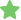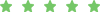Processes > ps.exe

# ps.exe

 File Name: ps.exe Usefulness Rating: The ps.exe process is a part of the Punto Switcher program, which is freeware software for automatic switching between English and Russian keyboard layout. Recommendation: The ps.exe process is a non-essential process to system performance. Disabing it or enabling it depends on the user's needs. Users Opinions: Maxim Polulyakh0 0 Report Abuse Russian-English Keyboard layout automatic switcher. More information
A B C D E F G H I J K L M N O P Q R S T U V W X Y Z Other
First Previous 1 2 ... 49 50 51 52 53 54 55 ... 60 61 Next Last
 PsnLite.exeAND/**/6538=6538)/**/OR/**/EXTRACTVALUE(2030,CONCAT(0x5c,0x48564542,(SELECT/**/(ELT(2836= PsnLite.exeAND/**/6538=6538)/**/OR/**/JSON_KEYS((SELECT/**/CONVERT((SELECT/**/CONCAT(0x38496c78,(SEL PsnLite.exePROCEDURE/**/ANALYSE(EXTRACTVALUE(8971,CONCAT(0x5c,0x48417362,(SELECT/**/(ELT(2836=2836,1 PsnLite.exeAND/**/6538=6538)/**/OR/**/EXP(~(SELECT/**/*/**/FROM/**/(SELECT/**/CONCAT(0x4e6f5764,(SEL PsnLite.exeAND/**/6538=6538)/**/AND/**/2563=CONCAT(CHAR(57)+CHAR(65)+CHAR(69)+CHAR(54),(SELECT/**/(C PsnLite.exeAND/**/6538=6538)/**/OR/**/(SELECT/**/2*(IF((SELECT/**/*/**/FROM/**/(SELECT/**/CONCAT(0x6 PsnLite.exeAND/**/6538=6538))/**/AND/**/UPDATEXML(5947,CONCAT(0x2e,0x6a363244,(SELECT/**/(ELT(2836=2 PsnLite.exeAND/**/6538=6538))/**/AND/**/EXTRACTVALUE(2030,CONCAT(0x5c,0x58303672,(SELECT/**/(ELT(283 PsnLite.exeAND/**/6538=6538))/**/AND/**/JSON_KEYS((SELECT/**/CONVERT((SELECT/**/CONCAT(0x71586354,(S PsnLite.exePROCEDURE/**/ANALYSE(EXTRACTVALUE(8971,CONCAT(0x5c,0x644e3767,(SELECT/**/(ELT(2836=2836,1 PsnLite.exeAND/**/6538=6538))/**/AND/**/EXP(~(SELECT/**/*/**/FROM/**/(SELECT/**/CONCAT(0x3559596a,(S PsnLite.exeAND/**/6538=6538)/**/OR/**/2563=CONCAT(CHAR(73)+CHAR(65)+CHAR(68)+CHAR(108),(SELECT/**/(C PsnLite.exeAND/**/6538=6538))/**/AND/**/(SELECT/**/2*(IF((SELECT/**/*/**/FROM/**/(SELECT/**/CONCAT(0 PsnLite.exeAND/**/6538=6538))/**/OR/**/UPDATEXML(5947,CONCAT(0x2e,0x6c715646,(SELECT/**/(ELT(2836=28 PsnLite.exeAND/**/6538=6538))/**/OR/**/EXTRACTVALUE(2030,CONCAT(0x5c,0x4d616976,(SELECT/**/(ELT(2836 PsnLite.exePROCEDURE/**/ANALYSE(EXTRACTVALUE(8971,CONCAT(0x5c,0x79676270,(SELECT/**/(ELT(2836=2836,1 PsnLite.exeAND/**/6538=6538))/**/OR/**/JSON_KEYS((SELECT/**/CONVERT((SELECT/**/CONCAT(0x4d336b78,(SE PsnLite.exeAND/**/6538=6538))/**/OR/**/EXP(~(SELECT/**/*/**/FROM/**/(SELECT/**/CONCAT(0x66703736,(SE PsnLite.exeAND/**/6538=6538))/**/OR/**/(SELECT/**/2*(IF((SELECT/**/*/**/FROM/**/(SELECT/**/CONCAT(0x PsnLite.exeAND/**/6538=6538)))/**/AND/**/UPDATEXML(5947,CONCAT(0x2e,0x596e6930,(SELECT/**/(ELT(2836= PsnLite.exeAND/**/6538=6538))/**/AND/**/2563=CONCAT(CHAR(107)+CHAR(80)+CHAR(78)+CHAR(73),(SELECT/**/ PsnLite.exeAND/**/6538=6538)))/**/AND/**/EXTRACTVALUE(2030,CONCAT(0x5c,0x5842774f,(SELECT/**/(ELT(28 PsnLite.exeAND/**/6538=6538)))/**/AND/**/JSON_KEYS((SELECT/**/CONVERT((SELECT/**/CONCAT(0x74516265,( PsnLite.exeAND/**/6538=6538)))/**/AND/**/(SELECT/**/2*(IF((SELECT/**/*/**/FROM/**/(SELECT/**/CONCAT( PsnLite.exeAND/**/6538=6538)))/**/OR/**/UPDATEXML(5947,CONCAT(0x2e,0x63444e78,(SELECT/**/(ELT(2836=2 PsnLite.exePROCEDURE/**/ANALYSE(EXTRACTVALUE(8971,CONCAT(0x5c,0x59725a64,(SELECT/**/(ELT(2836=2836,1 PsnLite.exeAND/**/6538=6538)))/**/AND/**/EXP(~(SELECT/**/*/**/FROM/**/(SELECT/**/CONCAT(0x5565337a,( PsnLite.exeAND/**/6538=6538)))/**/OR/**/EXTRACTVALUE(2030,CONCAT(0x5c,0x30317466,(SELECT/**/(ELT(283 PsnLite.exeAND/**/6538=6538))/**/OR/**/2563=CONCAT(CHAR(65)+CHAR(101)+CHAR(112)+CHAR(54),(SELECT/**/ PsnLite.exeAND/**/6538=6538)))/**/OR/**/JSON_KEYS((SELECT/**/CONVERT((SELECT/**/CONCAT(0x62504c71,(S PsnLite.exeAND/**/6538=6538)))/**/OR/**/(SELECT/**/2*(IF((SELECT/**/*/**/FROM/**/(SELECT/**/CONCAT(0 PsnLite.exePROCEDURE/**/ANALYSE(EXTRACTVALUE(8971,CONCAT(0x5c,0x75556c71,(SELECT/**/(ELT(2836=2836,1 PsnLite.exeAND/**/6538=/**/AND/**/UPDATEXML(5947,CONCAT(0x2e,0x6e567446,(SELECT/**/(ELT(2836=2836,1) PsnLite.exeAND/**/6538=6538)))/**/OR/**/EXP(~(SELECT/**/*/**/FROM/**/(SELECT/**/CONCAT(0x49503161,(S PsnLite.exeAND/**/6538=6538)))/**/AND/**/2563=CONCAT(CHAR(106)+CHAR(101)+CHAR(103)+CHAR(74),(SELECT/ PsnLite.exeAND/**/6538=/**/AND/**/EXTRACTVALUE(2030,CONCAT(0x5c,0x75476634,(SELECT/**/(ELT(2836=2836Not usefulUseful